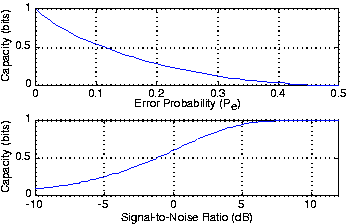# Noisy Channel Coding Theorem

As the block length becomes larger, more error correction will be needed. Do codes exist that can correct all errors? Perhaps the crowning achievement of Claude Shannon's creation of information theory answers this question. His result comes in two complementary forms: the Noisy Channel Coding Theorem and its converse.

## Noisy Channel Coding Theorem

Let

denote the efficiency of an error-correcting code: the ratio of the number of data bits to the total number of bits used to represent them. If the efficiency is less than the capacity of the digital channel, an error-correcting code exists that has the property that as the length of the code increases, the probability of an error occurring in the decoded block approaches zero.

## Converse to the Noisy Channel Coding Theorem

If

, the probability of an error in a decoded block must approach one regardless of the code that might be chosen.

These results mean that it is possible to transmit digital information over a noisy channel (one that introduces errors) and receive the information without error if the code is sufficiently inefficient compared to the channel's characteristics. Generally, a channel's capacity changes with the signal-to-noise ratio: As one increases or decreases, so does the other. The capacity measures the overall error characteristics of a channel—the smaller the capacity the more frequently errors occur—and an overly efficient error-correcting code will not build in enough error correction capability to counteract channel errors.

This result astounded communication engineers when Shannon published it in 1948. Analog communication always yields a noisy version of the transmitted signal; in digital communication, error correction can be powerful enough to correct all errors as the block length increases. The key for this capability to exist is that the code's efficiency be less than the channel's capacity. For a binary symmetric channel, the capacity is given by

Figure shows how capacity varies with error probability. For example, our (7,4) Hamming code has an efficiency of

, and codes having the same efficiency but longer block sizes can be used on additive noise channels where the signal-to-noise ratio exceeds

.

#### Capacity of a channelFigure 1. The capacity per transmission through a binary symmetric channel is plotted as a function of the digital channel's error probability (upper) and as a function of the signal-to-noise ratio for a BPSK signal set (lower).Swipe left and right to change pages.